# Concept 5 – Angles

Introduction to Angles8-53. ANGLE RULERS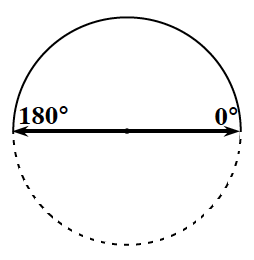How can you measure angles accurately using degrees?  In this activity, you will build a device (you can call it an “angle ruler”) to help you measure angles in degrees.

Your Task: Get a copy of the Lesson 8.3.1B Resource Page, which contains a diagram like the one at right.  Work with your team to find a way to fold and label the resource page multiple times to make it into an “angle ruler.”  The ruler is started with  and includes a mark for º180º.  Be sure to design your angle ruler so that you can measure common angles such as 30°45°60°90° and 135°.  Be prepared to explain your strategy.

8-55. Actually, “angle rulers” exist.  They are called protractors.
• Get a protractor from your teacher.  Compare it with the one that you made.  In what ways are they similar and in what ways are they different?

•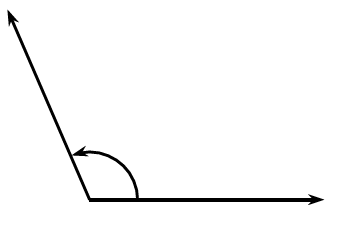Hunter used a protractor to measure the angle at right.  He got 60°.  Is his answer reasonable?  Explain why or why not.

• When measuring an angle with a protractor, is it necessary to line up one side of the angle with the º or º180º mark?  If you did not, how would you figure out what the measure of the angle was?  Explain.

8-56. Estimate the angles formed in the diagrams below without measuring.  Be ready to explain your estimates.
1.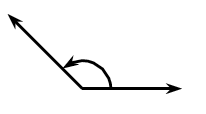1.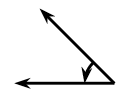1.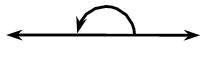1.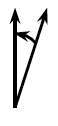8-57. Angles can be thought of as the result of a rotation or turn.  They can also help you to describe shapes.

Get a Lesson 8.3.1C Resource Page, which has larger diagrams of each of the figures below.  First estimate, then measure the indicated angles in degrees.

1.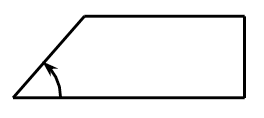1.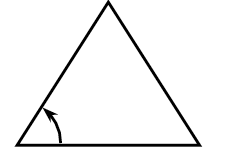1.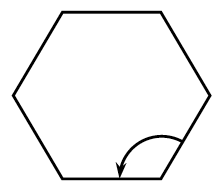Homework:

Classifying Angles8-66. In geometry, an angle is formed by two rays with a common endpoint.  A ray is a line that has an endpoint and extends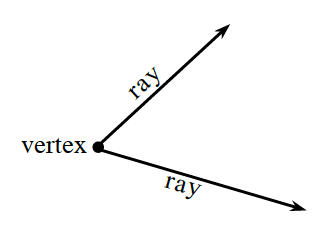infinitely in a particular direction.  The point where the two rays intersect is called the vertex of the angle.

One of the most common angles is a right angle.  A right angle measures º90º.  If an angle measures less than º90º, it is called an acute angle.  If an angle measures more than º90º but less than º180º, it is called an obtuse angle.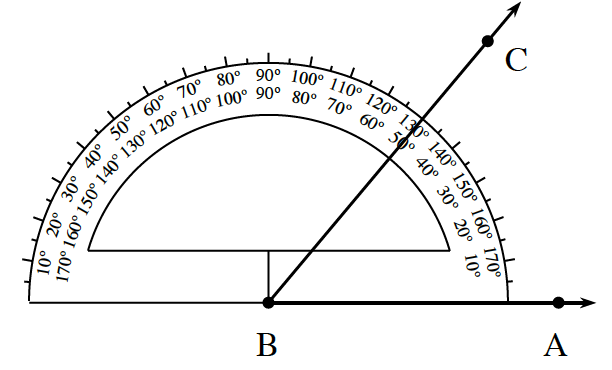Use a protractor to find the measure of each angle, and then classify it according to its measure.

1.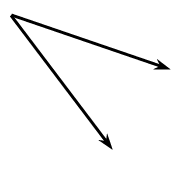1.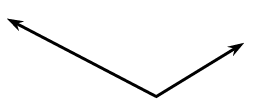1.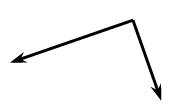1. A straight line is also called a straight angle.  Draw a straight angle on your paper.  What is the measure of a straight angle?

8-67. The diagrams below show some students’ use of protractors.  Explain what each student needs to do to find the correct angle measure.
1.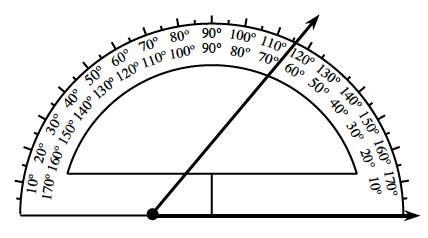1.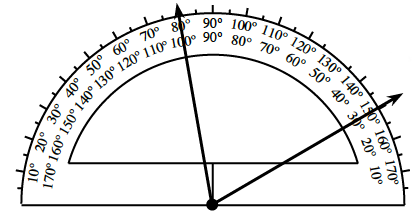1.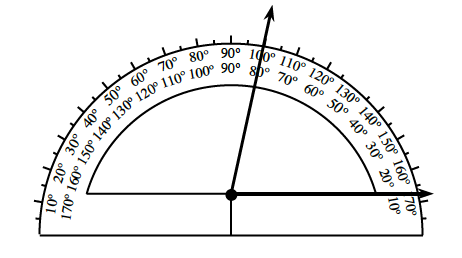1. m∠=60°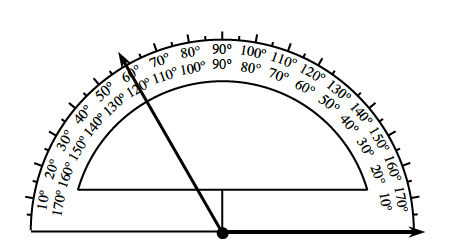8-68.

Complementary angles are two angles with a sum of º90º.

Supplementary angles are two angles with a sum of º180º.

1. Use your protractor to measure each of the angles below and determine which angles are complementary and which angles are supplementary.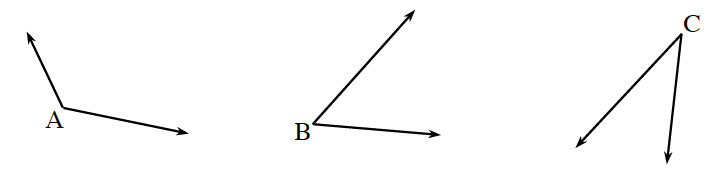2. With your team, come up with a strategy for remembering the definitions of complementary and supplementary.

Angles that share that same vertex and one side are referred to as adjacent angles.  “Adjacent” in the English language means “next to.”  For two angles to be adjacent, they must satisfy the following three conditions:

1. The two angles must have a common (shared or same) side.

2. They must have a common vertex (a common starting point for all sides).

3. They can have no interior points in common (no overlap is permitted).  The common side must be between the two angles.

Copy each of the figures below onto your paper.  Determine which pairs of angles are adjacent and which are not by checking to see if each angle pair satisfies all three conditions.  Write “yes” or “no” next to each figure.  If you write “no,” give a short explanation.

1.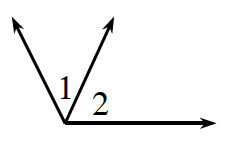1.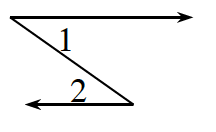1.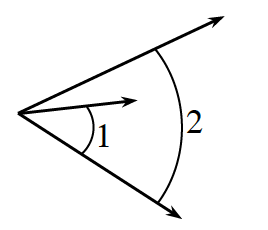1.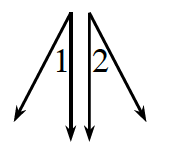1.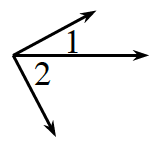1.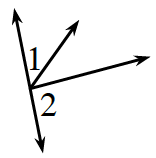1.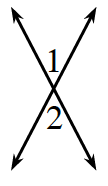1.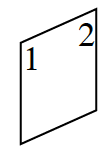1.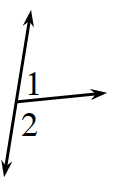8-70. VERTICAL ANGLES

Angles formed by two intersecting lines that are not adjacent are called vertical angles.  In the diagram below, angles 1 and 2 are vertical angles.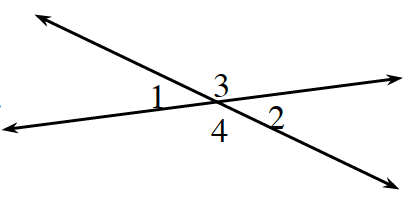1. What can you say about angles 3 and 4?

2. Use your protractor to measure each of the four angles.  What do you notice?

3. Draw a pair of vertical angles on your paper.  Each team member should draw angles with different measures.  Measure your vertical angles.  What do you think is true about the measures of vertical angles?

8-71. Find the measure of the missing angles.
1. ∠1=75°∠2=?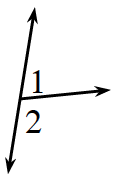1. ∠1=55°∠2=?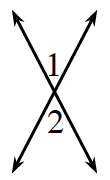1. ∠1 and ∠2 are complementary

∠1=27°∠2=?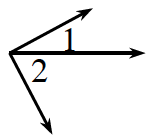1. ∠1 and ∠2 are supplementary

∠1=30°∠2=?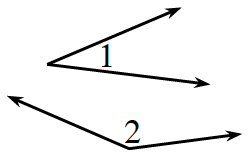8-72. The problems below describe other situations where some angles have been put together to create larger angles.  For each problem below, write an equation to represent the situation and then answer the question.
1. Three angles that all have the same measure and one 36° angle combine to make a 90° angle, as shown in the diagram at right.  What is the measure of each of the three unmarked angles?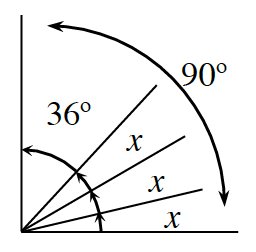1. Additional Challenge: One angle and two other angles that are each half as large as the first combine to create an angle that measures 140°, as shown in the diagram at right.  What are the measures of each of the angles?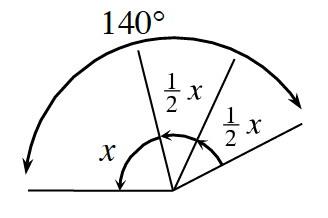8-73. Additional Challenge: Determine the size of all of the angles labeled with variables.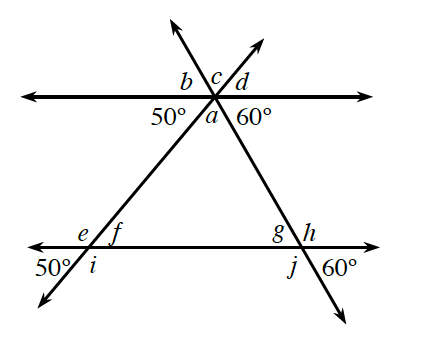Homework: